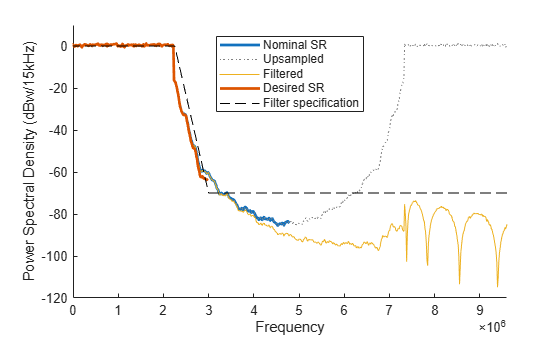This example shows the internal resampling filter design of the OFDM functions `nrOFDMModulate`, `nrOFDMInfo`, and `nrOFDMDemodulate`. These functions enable you to specify the OFDM sample rate and fast Fourier transform (FFT) size by using the `SampleRate` and `Nfft` name-value input arguments. When the specified sample rate and the nominal sample rate, corresponding to the FFT size, do not match, the OFDM functions resample the waveform using an internal multirate FIR filter.

### OFDM Sample Rate and Nominal Sample Rate

The value that you set for the `SampleRate` input determines the sample rate of the waveform.

The nominal sample rate corresponding to the FFT size used in the OFDM modulation, ${\mathit{FFT}}_{\mathrm{SR}}$, is equal to `Nfft*carrier.SubcarrierSpacing*1000`, where `carrier` is the input argument of the function call that specifies the carrier configuration.

When the specified sample rate and the nominal sample rate do not match, the OFDM functions resample the waveform using an internal resampling filter. Setting the sample rate to the nominal sample rate does not result in resampling during OFDM modulation.

### OFDM Modulation without Resampling

Create a carrier configuration object with 25 resource blocks.

```carrier = nrCarrierConfig; carrier.NSizeGrid = 25;```

Get OFDM information.

`ofdmInfo = nrOFDMInfo(carrier);`

Display nominal sample rate and the sample rate returned in the OFDM information.

`ofdmInfo.Nfft*carrier.SubcarrierSpacing*1000`
```ans = 7680000 ```
`ofdmInfo.SampleRate`
```ans = 7680000 ```

Verify that specifying a sample rate of `7.68e6` does not result in resampling during OFDM modulation.

```grid = nrResourceGrid(carrier); grid(:) = nrSymbolModulate(randi([0 1],numel(grid)*2,1),'QPSK'); waveform1 = nrOFDMModulate(carrier,grid); waveform2 = nrOFDMModulate(carrier,grid,'SampleRate',7.68e6); isequal(waveform1,waveform2)```
```ans = logical 1 ```

### Filter Design

The resampling filter is a function of these two ratios.

• The ratio of the transmission bandwidth (${\mathit{TX}}_{\mathrm{BW}}$) to the nominal sample rate: ${\mathit{TX}}_{\mathrm{BW}}$ / ${\mathit{FFT}}_{\mathrm{SR}}$, where ${\mathit{TX}}_{\mathrm{BW}}$ is equal to `carrier.NSizeGrid*12*carrier.SubcarrierSpacing*1000`.

• The ratio of the nominal sample rate to the specified sample rate: ${\mathit{FFT}}_{\mathrm{SR}}$ / `SampleRate`.

To design the resampling filter, the OFDM functions call the `designMultirateFIR` function with these input arguments.

• Interpolation factor, `L`, equal to `SampleRate/g`, where `g =` `gcd`(${\mathit{FFT}}_{\mathrm{SR}}$,`SampleRate`). Because the resampling of the OFDM-modulated waveform is by a factor of `SampleRate/`${\mathit{FFT}}_{\mathrm{SR}}$, the resampling is costly if `SampleRate` and ${\mathit{FFT}}_{\mathrm{SR}}$ do not have large common factors.

• Decimation factor, `M`, equal to ${\mathit{FFT}}_{\mathrm{SR}}$`/g`.

• Transition width, `TW`, such that the transition band starts at $±$${\mathit{TX}}_{\mathrm{BW}}$ / 2 (that is, the transition band starts at the edge of the occupied bandwidth).

• Stopband attenuation, `Astop`, equal to 70 dB.

If `SampleRate` > ${\mathit{FFT}}_{\mathrm{SR}}$, then:

• `L` > `M` and the transition band stops at $±$${\mathit{FFT}}_{\mathrm{SR}}$ / 2 (corresponding to a normalized frequency of 1/`L`).

• The filter acts as an anti-imaging filter after upsampling by `L`.

If `SampleRate` < ${\mathit{FFT}}_{\mathrm{SR}}$, then:

• `M` > `L` and the transition band stops at $±$`SampleRate/2` (corresponding to a normalized frequency of 1/`M`).

• The filter acts as an anti-aliasing filter before downsampling by `M`.

### OFDM Modulation with Resampling

Create a carrier configuration object with 25 resource blocks.

```carrier = nrCarrierConfig; carrier.NSizeGrid = 25;```

Compute the transmission bandwidth.

`txBW = carrier.NSizeGrid*12*carrier.SubcarrierSpacing*1000`
```txBW = 4500000 ```

Specify a custom sample rate. Obtain the corresponding OFDM information and calculate the nominal sample rate for the default FFT size.

```SampleRate = 6e6; ofdmInfo = nrOFDMInfo(carrier,'SampleRate',SampleRate); Nfft = ofdmInfo.Nfft```
```Nfft = 640 ```
`fftSR = Nfft*carrier.SubcarrierSpacing*1000`
```fftSR = 9600000 ```

Calculate `g`, `L`, and `M`.

`g = gcd(fftSR,SampleRate)`
```g = 1200000 ```
`L = SampleRate/g`
```L = 5 ```
`M = fftSR/g`
```M = 8 ```

Plot the spectrum of the OFDM waveform before resampling by setting the sample rate to the nominal sample rate.

```figure; hold on; [f,S] = measureSpectrum(carrier,Nfft,fftSR,1); plot(f,S,'LineWidth',2);```

Plot the spectrum of the OFDM waveform after upsampling by `L`, before applying the filter.

```[fL,S] = measureSpectrum(carrier,Nfft,fftSR,L); hL = plot(fL,S,':');```

Plot the spectrum of the OFDM waveform after applying the filter.

```[f,S] = measureSpectrum(carrier,Nfft,fftSR*L,1); plot(f,S);```

Plot the spectrum of the final OFDM waveform after downsampling.

```[f,S] = measureSpectrum(carrier,Nfft,SampleRate,1); plot(f,S,'Color',hL.Color,'LineWidth',2); hL.Color = [0.5 0.5 0.5];```

Overlay the filter design specification.

```fStop = fftSR/2*L/max([L M]); aStop = -70.0; spec = [fL(1) aStop; -fStop aStop; -txBW/2 0; txBW/2 0; fStop aStop; fL(end) aStop]; plot(spec(:,1),spec(:,2),'k--'); legend('Nominal SR','Upsampled','Filtered','Desired SR','Filter specification','Location','north'); xlabel('Frequency'); ylabel(['Power Spectral Density (dBw/' num2str(carrier.SubcarrierSpacing) 'kHz)']); axis([0 fftSR -120 10]);```### Local Function

```function [f,S] = measureSpectrum(carrier,Nfft,SampleRate,L) % Subcarrier spacing in Hz SCS = carrier.SubcarrierSpacing*1e3; % Set up spectrum estimator spectrumEstimator = dsp.SpectrumEstimator; spectrumEstimator.SampleRate = SampleRate; spectrumEstimator.AveragingMethod = 'Exponential'; spectrumEstimator.ForgettingFactor = 0.99; spectrumEstimator.FrequencyRange = 'centered'; spectrumEstimator.PowerUnits = 'dBW'; spectrumEstimator.SpectrumType = 'Power density'; spectrumEstimator.FFTLengthSource = 'Property'; spectrumEstimator.FFTLength = floor(SampleRate*L/SCS); % For 100 slots rs = RandStream('mt19937ar','Seed',1); for nSlot = 0:99 % Create a slot grid filled with QPSK symbols and OFDM modulate grid = nrResourceGrid(carrier); grid(:) = nrSymbolModulate(rs.randi([0 1],numel(grid)*2,1),'QPSK'); waveform = nrOFDMModulate(carrier,grid,'Nfft',Nfft,'SampleRate',SampleRate); waveform = waveform * Nfft; % Apply interpolation if required if (L>1) T = size(waveform,1); waveform = reshape([waveform.'; zeros(L-1,T)],[],1)*sqrt(L); end % Measure the spectrum S = spectrumEstimator(waveform); end % Create frequency axis N = spectrumEstimator.FFTLength; f = (-N/2:(N/2 - 1))*SCS; % Translate from dBw/Hz to dBW/SCS S = S + 10*log10(SCS); end```